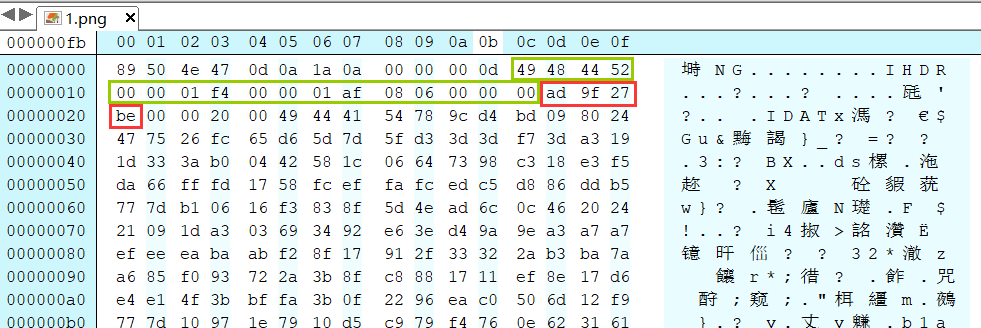Python3 修复PNG图片的宽高 CRC爆破• 累计撰写 57 篇文章
• 累计收到 1 条评论

# Python3 修复PNG图片的宽高 CRC爆破2020-03-06 / 0 评论 / 2,253 阅读 / 正在检测是否收录...

``````import binascii
import struct
import sys

file = input("图片地址：")
data = bytearray(fr[0x0c:0x1d])
crc32key = eval('0x'+str(binascii.b2a_hex(fr[0x1d:0x21]))[2:-1])
#原来的代码: crc32key = eval(str(fr[29:33]).replace('\\x','').replace("b'",'0x').replace("'",''))
n = 4095
for w in range(n):
width = bytearray(struct.pack('>i', w))
for h in range(n):
height = bytearray(struct.pack('>i', h))
for x in range(4):
data[x+4] = width[x]
data[x+8] = height[x]
crc32result = binascii.crc32(data) & 0xffffffff
if crc32result == crc32key:
print(width,height)
newpic = bytearray(fr)
for x in range(4):
newpic[x+16] = width[x]
newpic[x+20] = height[x]
fw = open(file+'.png','wb')
fw.write(newpic)
fw.close
sys.exit()``````binascii.b2a_hex()函数是把bytes转成hex, 如果原来的数据是`b'\x61\x62\x63\x90'`, 用这个函数后就变成了`b'61626390'` 这样再转成字符串就可以了

13